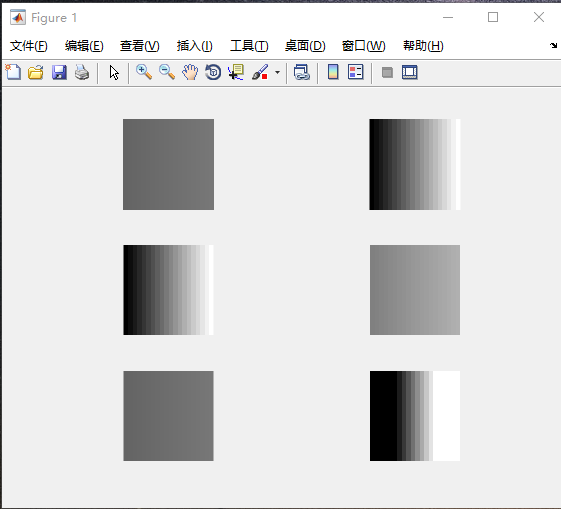MATLAB imshow()函数

imshow(I,[low high]) 显示灰度图像 I，以二元素向量 [low high] 形式指定显示范围。

A=im2uint8(zeros(r,c))
for r=1:100
for c=1:100
A(r,c)=  100+ floor( (c-1)/5);
end
end
A(1,:)%A中灰度值的范围为[100,119]
subplot(3,2,1),imshow(A);  %
subplot(3,2,2),imshow(A,[]); %相当于imshow[min(A(:)),max(A(:))],将[min(A(:)),max(A(:))]映射到[0,255]
subplot(3,2,3),imshow(A,[100,119]);  %与imshow(A,[])效果一样
subplot(3,2,4),imshow(A,[50,150]);
subplot(3,2,5),imshow(A,[110,110]);
subplot(3,2,6),imshow(A,[105,114]);